College Physics : Lenses

Example Questions

Example Question #1 : Optics

For most people, the nearest distance that objects can be located away from the eye and still seen clearly is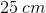. This is referred to as the near point; if the object comes any closer, the object cannot be seen clearly. Suppose that a person who needs glasses cannot see objects clearly if they are closer than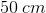from the eye; that is to say, their near point is. A lens with what refractive power is needed in order to correct this person's vision to bring their near point to?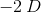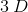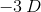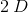Explanation:

For this question, we're given the definition of near point. We're told what the near point is in the average person, and also the near point for a certain person who can't see well and needs glasses. We're asked to find the refractive power of a lens that will bring this individual's near point to the average, healthy value.

As was stated in the question stem, the near point is the closest distance of an object from the eye where that object can still be seen clearly. In the question, we're told that the normal value for this is. Moreover, a person with a near point ofmeans that the object will need to be twice as far away, and no closer, to be seen clearly. Thus, in order to correct for this, a lens will be needed.

The idea is to be able to make the individual see things clearly when objects are locatedaway. To accomplish this, a lens will need to diffract the light coming from an objectaway. This diffracted light will then need to form an imageaway, which is where this particular individual's near point is.

With this information in hand, we can use the lens-makers equation to solve for the refractive power of the lens.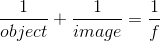We know that the object will be located a distance ofaway. Moreover, the image will need to form at a distance ofaway. However, since the image is forming on the same side of the lens that the object is located, the image will be virtual. Thus, the value used in the equation will be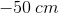.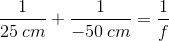Also, remember that to find refractive power, we'll need to have our units be in meters.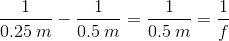Furthermore, recall that refractive power is equal to the inverse of focal length.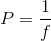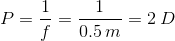Hence, the power of the refractive lens will need to bediopters to correct this person's near point.

Example Question #79 : College Physics

A convex lens connected to a projector projects an image onto a board of an object that is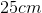away from the lens. If the object is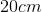tall and the inverted image is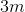tall, what is the focal length of the projector lens?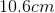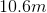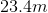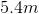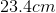Explanation:

To solve this one will require two equations. First, to find focal length, you will need the lens equation.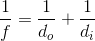But we are only given the object distance, not the image distance. So to find that we need to know the relationship between magnification and object and image distances.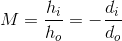Plug in the quantities we know.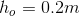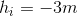(remember it was inverted)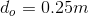. Then solve for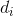which is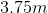. So the projector would sit that far from the board.

Now finally we can find the focal length of this lens.

Plugging in: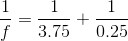Solving forgives a focal length of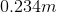orExample Question #80 : College Physics

An object located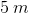away from a convex lens whose focal point is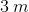produces an image located at what distance away from the lens?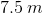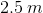Explanation:

For this question, we'll need to use the thin lens equation in order to determine the image distance from the lens.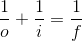Because this is a convex lens, the refracted light will form an image on the side of the lens where light is expected to go (real image). Thus, we know that the focal length should be a positive value. Moreover, the object distance is also positive.

Rearranging the above equation, we can isolate the term for image distance.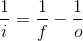Then we can plug in the values given to us in the question stem.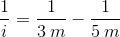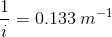And finally we take the inverse of this value to arrive at the correct answer.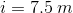All College Physics Resources